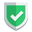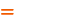我们相信：世界是美好的，你是我也是。平行空间的世界里面，不同版本的生活也在继续...

## 基本用法`clone \$obj`及`__clone()`

``````class class1 {
public function __clone() {
}
}
\$obj = new class1();
\$obj2= clone \$obj;
\$obj3= clone new class1();``````

``\$obj3= clone new class1();``

## 强化训练

``````class class1 {
public \$attr1;
private \$attr2;
private \$attr3;
public function __construct(\$a1 = "", \$a2 = "") {
\$this->attr1 = \$a1;
\$this->attr2 = \$a2;
\$this->attr3 = "这个是自动化初始值";
}
public function __clone() {
\$this->attr3 = "这个是clone时自动生成的值";
}
}
echo "<pre>";
\$c1 = new class1("attr1初始值", "attr2初始值");
\$c2 = \$c1;
\$c3 = clone \$c1;
\$c1->attr1 = "attr1被修改了";
print_r(\$c1);
print_r(\$c2);
print_r(\$c3);
echo "</pre>";``````

newsn.net：这里是【评论】可见内容

## 错误的使用方法

``````\$d1=["a","b","c"];
\$d2=clone \$d1;``````

``````\$a1 = ["a", "b", "c"];
\$a2 = \$a1;
\$a3 = array_merge([], \$a1);
\$a1 = "aa";
echo "<pre>";
print_r(\$a1);
print_r(\$a2);
print_r(\$a3);``````

newsn.net：这里是【评论】可见内容

## 结论如果本文对您有帮助，或者节约了您的时间，欢迎打赏瓶饮料，建立下友谊关系。本博客不欢迎：各种镜像采集行为。请尊重原创文章内容，转载请保留作者链接。【福利】 腾讯云最新爆款活动！1核2G云服务器首年50元！【源码】本文代码片段及相关软件，请点此获取更多信息【绝密】秘籍文章入口，仅传授于有缘之人php    clone